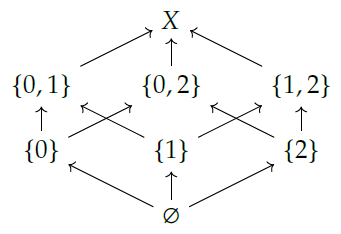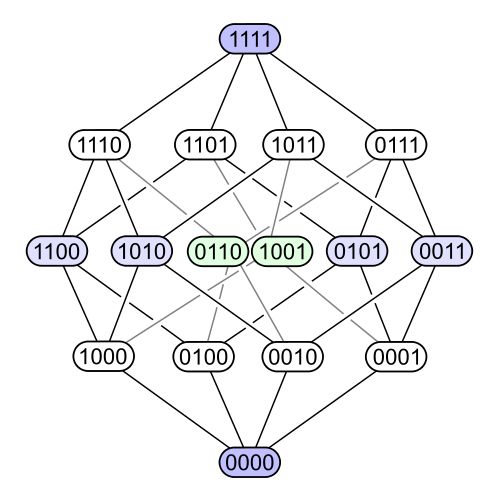#### Howdy, Stranger!

It looks like you're new here. If you want to get involved, click one of these buttons!

Options

# Exercise 41 - Chapter 1

edited June 2018

Draw the Hasse diagrams for $$P(\emptyset)$$, $$P\{1\}$$, and $$P\{1, 2\}$$ where $$P(X)$$ is the power set of $$X$$, e.g.

$$P\{1, 2\} = \{∅, \{1\}, \{2\}, \{1,2\}\}.$$

• Options
1.
edited April 2018

Hello, I was doing this exercise. On page 13 of the book, there is a hint: "a powerset of a finite set, say P{0,1,2,...,n}, always looks like a cube of dimension n." Example 1.36 shows a cube of dim. 3 for P{0,1,2}.

In the exercise, I guess that the Hasse diagram of P{1,2} is a square -- e.g., as a cube can be seen in Flatland -- the Hasse diagram of P{1} is just an arrow between two points -- e.g., as a square can be seen in Lineland -- and the Hasse diagram of P{∅} is just the ∅ itself with no arrows -- e.g., as a line looks like in Pointland. The number of objects is a power of 2, where the exponent of 2 is the number of dimensions. The Hasse diagram of P{1,2}, if I did not make any mistakes, looks like a square with arrows from ∅ to {1}, from ∅ to {2}, from {1} to {1,2}, and from {2} to {1,2}. Please, let me know if you think this is correct. I'm new in this course, and it's nice to learn and share ideas.

Comment Source:Hello, I was doing this exercise. On page 13 of the book, there is a hint: "a powerset of a finite set, say P{0,1,2,...,n}, always looks like a cube of dimension n." Example 1.36 shows a cube of dim. 3 for P{0,1,2}. In the exercise, I guess that the Hasse diagram of P{1,2} is a square -- e.g., as a cube can be seen in Flatland -- the Hasse diagram of P{1} is just an arrow between two points -- e.g., as a square can be seen in Lineland -- and the Hasse diagram of P{∅} is just the ∅ itself with no arrows -- e.g., as a line looks like in Pointland. The number of objects is a power of 2, where the exponent of 2 is the number of dimensions. The Hasse diagram of P{1,2}, if I did not make any mistakes, looks like a square with arrows from ∅ to {1}, from ∅ to {2}, from {1} to {1,2}, and from {2} to {1,2}. Please, let me know if you think this is correct. I'm new in this course, and it's nice to learn and share ideas.
• Options
2.

That sounds about right! In fact, the Hasse diagram for $$\mathcal{P}\{0, 1, 2\}$$ in the book contains all three of these. As you noticed, this is a cube -- and one of the faces of this cube is a square giving the Hasse diagram for $$\mathcal{P}\{1, 2\}$$! One of the edges on this face gives the Hasse diagram for $$\mathcal{P}\{1\}$$, and the bottom-most vertex gives the Hasse diagram for $$\mathcal{P}\emptyset$$.

Comment Source:That sounds about right! In fact, the Hasse diagram for \$$\mathcal{P}\\{0, 1, 2\\}\$$ in the book contains all three of these. As you noticed, this is a cube -- and one of the faces of this cube is a square giving the Hasse diagram for \$$\mathcal{P}\\{1, 2\\}\$$! One of the edges on this face gives the Hasse diagram for \$$\mathcal{P}\\{1\\}\$$, and the bottom-most vertex gives the Hasse diagram for \$$\mathcal{P}\emptyset\$$.
• Options
3.
edited April 2018

I think you're right, Maria! Here is a picture of $$P(\{0,1,2\})$$:and here is a picture of $$P(\{0,1,2,3\})$$, drawn in a different style, where we write a bit to say whether each element is in or out of the subset:You can see that this is a 4-dimensional cube.

Comment Source:I think you're right, Maria! Here is a picture of \$$P(\\{0,1,2\\})\$$: <center><img src = "http://math.ucr.edu/home/baez/mathematical/7_sketches/P3_hasse_diagram.png"></center> and here is a picture of \$$P(\\{0,1,2,3\\})\$$, drawn in a different style, where we write a bit to say whether each element is in or out of the subset: <center><img src = "http://math.ucr.edu/home/baez/mathematical/7_sketches/P4_hasse_diagram.png"></center> You can see that this is a 4-dimensional cube.
• Options
4.

Thank you! The 4-dimensional cube looks great. It's nice to see how geometry arises so naturally. Personally, I better understand math when I can come up with some visualization strategy.

Comment Source:Thank you! The 4-dimensional cube looks great. It's nice to see how geometry arises so naturally. Personally, I better understand math when I can come up with some visualization strategy.